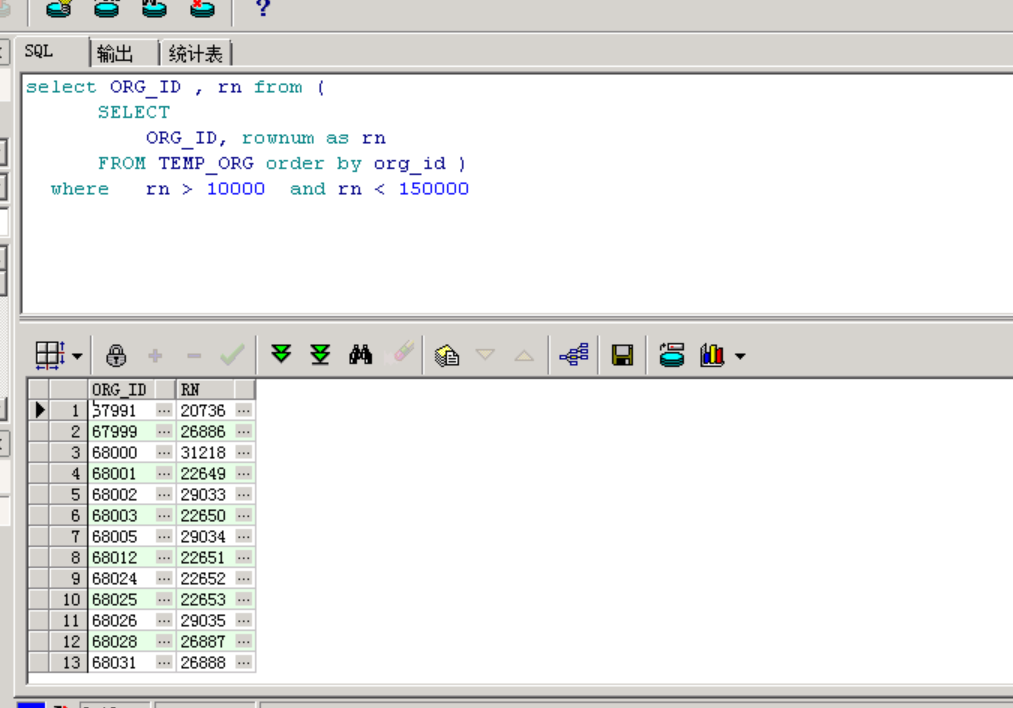ORACLE大数据排序order BY分页问题select ORG_ID , rn from (
SELECT
ORG_ID, rownum as rn
FROM TEMP_ORG order by org_id )
where rn > 10000 and rn < 150000

rn变成乱序的了，这到底是什么原因？还有就是分页查询应该order by rn ？

9个回答

select o.ORG_ID, o.rn
from (select i.ORG_ID, rownum as rn
from (SELECT ORG_ID FROM TEMP_ORG order by org_id) i /*内层进行*/
where rownum < 150000) o /*第二层取最大*/
where o.rn > 10000 /*第一层取最小*/

order by 提到外面一层试试3 年多之前 回复

rownum是动态加载的，有当前行就加载到当前行，在使用时只能用rownum<=?不能使用>,例如只有10行记录，rownum取不到11的。所以当你把rownum筛选来在根据他进行筛选出就会不一致

select b.* from
(select rownum rn,a.* from
(select teo.* from TEMP_ORG teo orser by org_id) a) b
where b.rn < 150000 and b.rn > 10000;

``````    先排序，在分页，然后使用条件查询
``````
``````
``````

select o.ORG_ID, o.rn
from (select i.ORG_ID, rownum as rn
from (SELECT ORG_ID FROM TEMP_ORG order by org_id) i
where rownum < 150000) o
where o.rn > 10000

ASC是什么意思求解，
order by 是取出数据后再排，也就是说id对应的rn生成后再以id排序，如果id不是自增那rn就会乱3 年多之前 回复

select *
from (select ORG_ID, rownum as rn
from (SELECT ORG_ID FROM TEMP_ORG order by org_id))
where rn > 10000
and rn < 150000

`````` 试试这样查询
``````